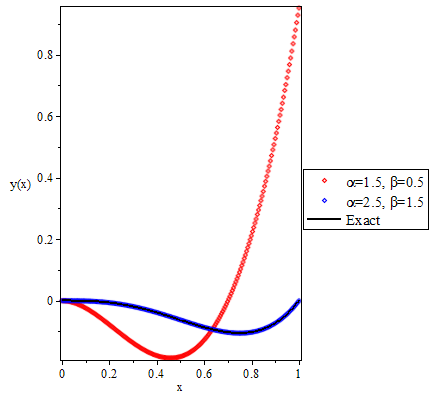# Perturbed Collocation Method For Solving Singular Multi-order Fractional Differential Equations of Lane-Emden Type

## Authors

• O. A. Uwaheren Department of Mathematics, University of Ilorin, Ilorin, Nigeria
• A. F. Adebisi Department of Mathematics, Osun State University, Oshogbo, Nigeria.
• O. A. Taiwo Department of Mathematics, University of Ilorin, Nigeria.

## Keywords:

Perturbed Colocation Method, Legendre polynomial, Singular multi-order differential equations, Lane-Emden equations

## Abstract

In this work, a general class of multi-order fractional differential equations of Lane-Emden type is considered. Here, an assumed approximate solution is substituted into a slightly perturbed form of the general class and the resulting equation is collocated at equally spaced interior points to give a system of linear algebraic equations which are then solved by suitable computer software; Maple 18.

Dimensions

S. Abbasbandy. “Application of He’s homotopy perturbation method for Laplace transform”, Chaos Solution & Fractals 30 (2006) 1206. DOI: https://doi.org/10.1016/j.chaos.2005.08.178

M. Dehghan & F. Shakeri. “Solution of an integro-differential equation arising in Oscillating Magnetic Fields using He’s Homotopy Perturbation Method”, PIER 78 (2008) 361. DOI: https://doi.org/10.2528/PIER07090403

Z. Odibat & S. Momani. “Modified homotopy perturbation method: application to quadratic riccati differential equation of fractional order”, Chaos, Solution and Fractals 36 (2008) 167. DOI: https://doi.org/10.1016/j.chaos.2006.06.041

I. K. Omar. “Variational iteration method for solving multi fractional integrodifferential equations”, Iraqi Journal of Science 55 (2014) 1086.

A. Yildirim & T. Qzis. “Solution of singular IVPs of Lane-Emden type by variational iteration method”, Nonlinear Analysis: Theory, Method & Applications 70 (2009) 2480. doi.org/10.1016/j.na.2008.03.012 DOI: https://doi.org/10.1016/j.na.2008.03.012

Y. Yang. “Solving a nonlinear multi-order fractional differential equation using Legendre pseudo-spectral method”, Journal of Applied Mathematics 4 (2013) 113. DOI: https://doi.org/10.4236/am.2013.41020

L. Jianping, L. Xia, X. Liyong & S. Yufa. “Solution of fractional differential equations by Bernstein polynomials method”, Journal of Convergence Information Technolgy 7 (2012) 820.

A. Barzkar, M. Oshagh, P. Assari & M. Mehrpouya. “Numerical solution of the nonlinear Fredholm integral equation and Fredholm integro-differential equation of second kind using Chebyshev wavelets”, World Applied Sciences Journal 18 (2012) 1774. doi.org/10.5829/idosi.wasj.2012.18.12.920.

Z. Avazzadeh & M. Heydari. “Chebyshev polynomials for solving two dimensional linear and nonlinear integral equations of the second kind”, Computational and Applied Mathematics 31 (2012) 127. DOI: https://doi.org/10.1590/S1807-03022012000100007

H. Cerdik-Yaslan & Z. Akyuz-Dascioglu. “Chebyshev polynomial solution of nonlinear Fredholm-Volterra integro-differential equation”, Journal of Arts Science 5 (2006) 89.

O. A. Uwaheren & O. A. Taiwo. “A collocation technique based on an orthogonal polynomial for solving multi fractional order integro differential equations”, Journal Mathematical Society of Nigeria (ABACUS) 43 (2016) 215.

M. Yigider. “The numerical method for solving differential equations of Lane-Emden type by Pade approximation”, Discrete Dynamics in Nature and Society (2011) 1. doi.org/10.1155/2011/479396 DOI: https://doi.org/10.1155/2011/479396

S. M. Mohammed & S. Norazak. “Numerical study of fractional differential equations of Lane-Emden type by method of collocation”, Applied Mathematics 3 (2012) 851. doi.org/10.4236/am.2012.38126. DOI: https://doi.org/10.4236/am.2012.38126

A. Verma & M. Kumar. “Numerical solution of Lane-Emden type equation using multilayer perceptron neural network method”, International Journal of Applied and Computational Mathematics 5 (2019) 141. link.springer.com DOI: https://doi.org/10.1007/s40819-019-0728-6

A. H. Bhrawy & S. A. Alofi. “A Jacob Gauss collocation method for solving nonlinear Lane-Emden type equation”, Communications in Nonlinear Science and Numerical Simulation 17 (201) 62. DOI: https://doi.org/10.1016/j.cnsns.2011.04.025

K. Parand, M. Dehgham, A. Rezaeia & S. Ghaderi. “An application algorithm for the solution of the nonlinear Lane-Emden type equations arising in astrophysics using Hermite functions collocation method”, Computer Physics Communications 181 (2010) 1096. doi.org/10.1016/j.cpc.2010.02.018

W. Al-Hayani, L. Alzubaidy & A. Entesar. “Solutions of singular IVPs of Lane-Emden type by homotopy analysis method with genetic algorithm”, Applied Mathematics and Information Sciences 11 (2019) 1. doi.org/10.18576/amis/paper2020-08-01

## How to Cite

Uwaheren, O. A., Adebisi, A. F., & Taiwo, O. A. . (2020). Perturbed Collocation Method For Solving Singular Multi-order Fractional Differential Equations of Lane-Emden Type. Journal of the Nigerian Society of Physical Sciences, 2(3), 141–148. https://doi.org/10.46481/jnsps.2020.69

## Section

Original Research